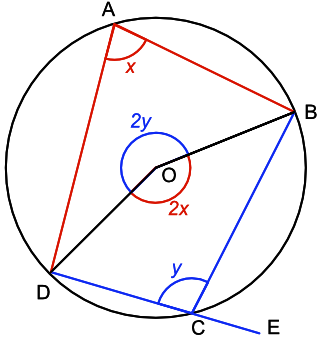## Circle Theorems - Cyclic Quadrilaterals

A quadrilateral where all four vertices touch the circumference of a circle is known as a cyclic quadrilateral.The angle at the centre of a circle is twice that of an angle at the circumference when subtended by the same arc. For arc D-A-B, let the angles be 2x and x respectively.

For the arc D-C-B, let the angles be 2y and y.

At the centre of the circle, 360 = 2(x +y), text(or) 180 = x + y

The exterior angle, ∠BCE, is 180 - y = x.

The exterior angle of a cyclic quadrilateral is equal to the opposite interior angle.

## Example 1

What is the value of the angle ∠DAB?180 - 102 = 78º